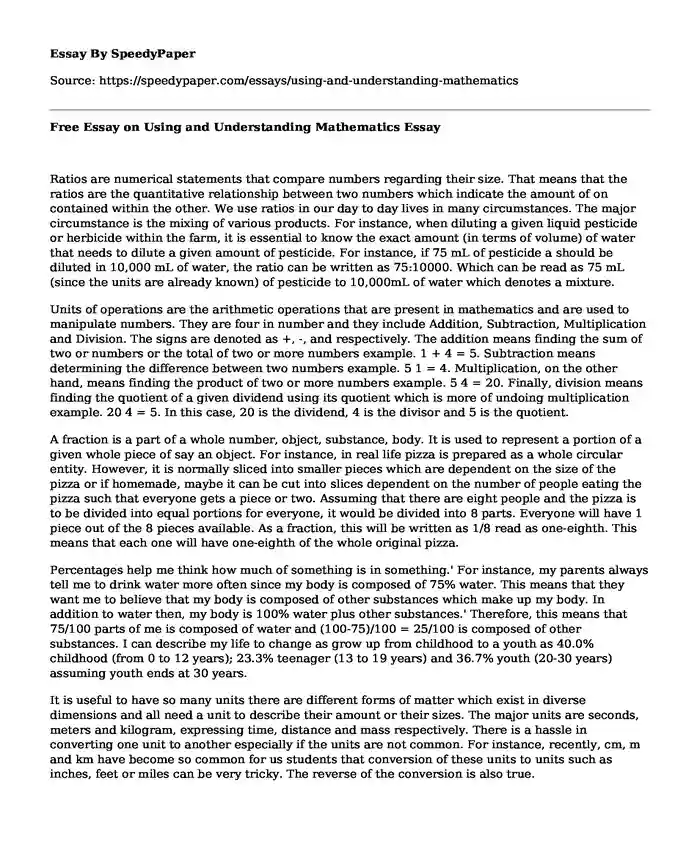# Free Essay on Using and Understanding Mathematics

Published: 2019-11-18 15:21:53Categories: Mathematics Pages: 3 Wordcount: 647 words
143 views

Ratios are numerical statements that compare numbers regarding their size. That means that the ratios are the quantitative relationship between two numbers which indicate the amount of on contained within the other. We use ratios in our day to day lives in many circumstances. The major circumstance is the mixing of various products. For instance, when diluting a given liquid pesticide or herbicide within the farm, it is essential to know the exact amount (in terms of volume) of water that needs to dilute a given amount of pesticide. For instance, if 75 mL of pesticide a should be diluted in 10,000 mL of water, the ratio can be written as 75:10000. Which can be read as 75 mL (since the units are already known) of pesticide to 10,000mL of water which denotes a mixture.

Is your time best spent reading someone else’s essay? Get a 100% original essay FROM A CERTIFIED WRITER!

Units of operations are the arithmetic operations that are present in mathematics and are used to manipulate numbers. They are four in number and they include Addition, Subtraction, Multiplication and Division. The signs are denoted as +, -, and respectively. The addition means finding the sum of two or numbers or the total of two or more numbers example. 1 + 4 = 5. Subtraction means determining the difference between two numbers example. 5 1 = 4. Multiplication, on the other hand, means finding the product of two or more numbers example. 5 4 = 20. Finally, division means finding the quotient of a given dividend using its quotient which is more of undoing multiplication example. 20 4 = 5. In this case, 20 is the dividend, 4 is the divisor and 5 is the quotient.

A fraction is a part of a whole number, object, substance, body. It is used to represent a portion of a given whole piece of say an object. For instance, in real life pizza is prepared as a whole circular entity. However, it is normally sliced into smaller pieces which are dependent on the size of the pizza or if homemade, maybe it can be cut into slices dependent on the number of people eating the pizza such that everyone gets a piece or two. Assuming that there are eight people and the pizza is to be divided into equal portions for everyone, it would be divided into 8 parts. Everyone will have 1 piece out of the 8 pieces available. As a fraction, this will be written as 1/8 read as one-eighth. This means that each one will have one-eighth of the whole original pizza.

Percentages help me think how much of something is in something.' For instance, my parents always tell me to drink water more often since my body is composed of 75% water. This means that they want me to believe that my body is composed of other substances which make up my body. In addition to water then, my body is 100% water plus other substances.' Therefore, this means that 75/100 parts of me is composed of water and (100-75)/100 = 25/100 is composed of other substances. I can describe my life to change as grow up from childhood to a youth as 40.0% childhood (from 0 to 12 years); 23.3% teenager (13 to 19 years) and 36.7% youth (20-30 years) assuming youth ends at 30 years.

It is useful to have so many units there are different forms of matter which exist in diverse dimensions and all need a unit to describe their amount or their sizes. The major units are seconds, meters and kilogram, expressing time, distance and mass respectively. There is a hassle in converting one unit to another especially if the units are not common. For instance, recently, cm, m and km have become so common for us students that conversion of these units to units such as inches, feet or miles can be very tricky. The reverse of the conversion is also true.

Free Essay on Using and Understanding Mathematics. (2019, Nov 18). Retrieved from https://speedypaper.com/essays/using-and-understanding-mathematics

Request Removal

If you are the original author of this essay and no longer wish to have it published on the SpeedyPaper website, please click below to request its removal:

Liked this essay sample but need an original one?

Hire a professional with VAST experience!